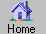Prev Next Index-> contents reference index search external Up-> CppAD Introduction CppAD-> Install Introduction AD ADFun preprocessor multi_thread utility ipopt_solve Example speed Appendix Introduction-> exp_2 exp_eps exp_apx.cpp Headings-> Purpose Preface ---..Algorithmic Differentiation ---..Forward Mode ---..Reverse Mode ---..Operation Count ---..Efficiency Outline Reference Contents

An Introduction by Example to Algorithmic Differentiation

Purpose
This is an introduction by example to Algorithmic Differentiation. Its purpose is to aid in understand what AD calculates, how the calculations are preformed, and the amount of computation and memory required for a forward or reverse sweep.

Preface

Algorithmic Differentiation
Algorithmic Differentiation (often referred to as Automatic Differentiation or just AD) uses the software representation of a function to obtain an efficient method for calculating its derivatives. These derivatives can be of arbitrary order and are analytic in nature (do not have any truncation error).

Forward Mode
A forward mode sweep computes the partial derivative of all the dependent variables with respect to one independent variable (or independent variable direction).

Reverse Mode
A reverse mode sweep computes the derivative of one dependent variable (or one dependent variable direction) with respect to all the independent variables.

Operation Count
The number of floating point operations for either a forward or reverse mode sweep is a small multiple of the number required to evaluate the original function. Thus, using reverse mode, you can evaluate the derivative of a scalar valued function with respect to thousands of variables in a small multiple of the work to evaluate the original function.

Efficiency
AD automatically takes advantage of the speed of your algorithmic representation of a function. For example, if you calculate a determinant using LU factorization, AD will use the LU representation for the derivative of the determinant (which is faster than using the definition of the determinant).

Outline
1. Demonstrate the use of CppAD to calculate derivatives of a polynomial: get_started.cpp .
2. Present two algorithms that approximate the exponential function. The first algorithm exp_2.hpp is simpler and does not include any logical variables or loops. The second algorithm exp_eps.hpp includes logical operations and a `while` loop. For each of these algorithms, do the following:
1. Define the mathematical function corresponding to the algorithm (exp_2 and exp_eps ).
2. Write out the floating point operation sequence, and corresponding values, that correspond to executing the algorithm for a specific input (exp_2_for0 and exp_eps_for0 ).
3. Compute a forward sweep derivative of the operation sequence (exp_2_for1 and exp_eps_for1 ).
4. Compute a reverse sweep derivative of the operation sequence (exp_2_rev1 and exp_eps_rev1 ).
5. Use CppAD to compute both a forward and reverse sweep of the operation sequence (exp_2_cppad and exp_eps_cppad ).
3. The program exp_apx.cpp runs all of the test routines that validate the calculations in the exp_2 and exp_eps presentation.

Reference
An in-depth review of AD theory and methods can be found in the book ```Evaluating Derivatives: Principles and Techniques of Algorithmic Differentiation ``` , Andreas Griewank, SIAM Frontiers in Applied Mathematics, 2000.

Contents
 exp_2 Second Order Exponential Approximation exp_eps An Epsilon Accurate Exponential Approximation exp_apx.cpp Correctness Tests For Exponential Approximation in Introduction

Input File: omh/introduction.omh